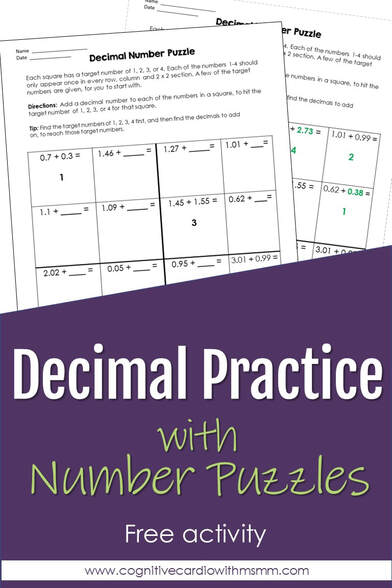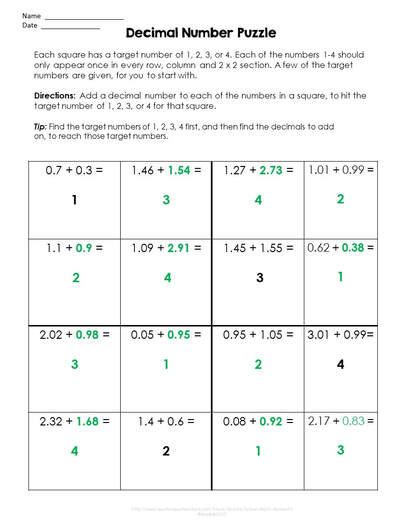# Decimal Operations Practice With Number Puzzles

Free Number Puzzle!Working with Decimal Operations
How much do your students love working with decimals??

Sometimes remembering decimal operations ‘rules’ can be difficult, but practicing with math puzzles can help!
Turning decimal operations practice into a fun, Sudoku-like puzzle is a great way to help upper elementary and middle school math students work their way through the different decimal operations.

Many of my students love solving Sudoku puzzles using whole numbers. When we replace the whole numbers with decimal problems, we’re able to use a logic puzzle that gives students a new way to practice!

Solving Number Puzzles
Sudoku puzzles are fun and interesting. In case you’ve never used them – they require students to problem solve, to make sure that every row, column and group of squares only has one of each number. Some students develop strategies when approaching a puzzle; some learn to use guess and check quite often:-)

When working with decimals in a Sudoku puzzle, students need to consider their target numbers, as well as how to complete the necessary operation. This makes decimal practice a bit more interesting and engaging than working on one problem after another on a worksheet.As with whole number Sudoku, I start decimal Sudoku with a few squares filled in, so students have a starting point. (If students have never tried Sudoku puzzles before, I recommend starting with a regular Sudoku puzzle, to teach students how they work.)

So far, I’ve only used a 4 X 4 grid, which makes figuring out the target numbers fairly easy. All of the squares have a decimal problem with a missing addend in them (in this example). Within each 2 x 2 section, there is one completed problem, with the target number of 1, 2, 3, or 4 already filled in. The object is for students to figure out the target number for each square and then find the missing decimal number in each individual square. Every row and column must each contain 1, 2, 3 and 4 as the answers to the decimal problems (these are the target numbers). Every 2 x 2 section must also contain 1, 2, 3 and 4.

For example, on the answer key shown here, you can see the target numbers of 1-4 in each row, column and section, and you can see the completed decimal problems. Everything in black (target numbers and decimal numbers) is provided for students. Everything in green is what the students must find (target numbers and missing decimal addends):

I’ve only used the addition problems (which actually requires them to subtract:-), but I plan to try the other operations as well and create larger puzzles (6 x 6 and maybe 9 x 9).

To make students really think things through, I may mix up the operations! For instance, in a 4 X 4 puzzle, the first column could be two multiplication problems and two addition problems. This should keep students paying close attention.

These could be great to complete on mini dry erase boards – easy to erase any guesses that don’t work out!

## EllieWelcome to Cognitive Cardio Math! I’m Ellie, a wife, mom, grandma, and dog ‘mom,’ and I’ve spent just about my whole life in school! With nearly 30 years in education, I’ve taught:

• All subject areas in 4th and 5th grades
• Math, ELA, and science in 6th grade (middle school)

I’ve been creating resources for teachers since 2012 and have worked in the elearning industry for about five years as well!